0

# Pandas数据分析：快速图表可视化各类操作详解+实例代码(二)

## 前言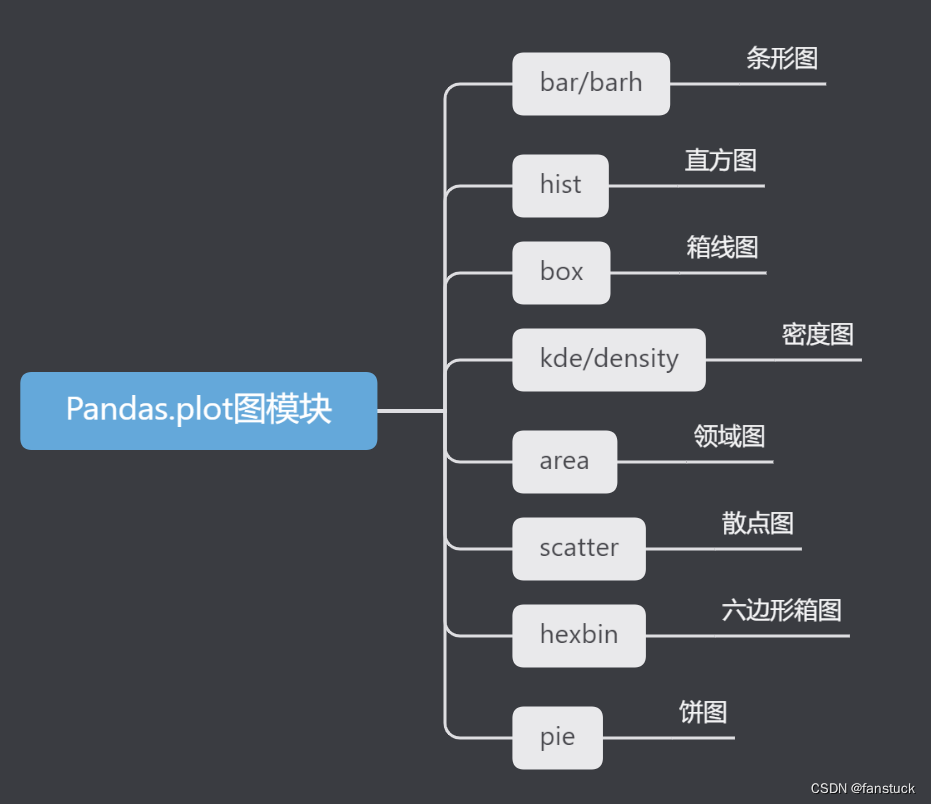Pandas数据分析：快速图表可视化各类操作详解+实例代码(一)

Pandas数据分析系列专栏已经更新了很久了，基本覆盖到使用pandas处理日常业务以及常规的数据分析方方面面的问题。从基础的数据结构逐步入门到处理各类数据以及专业的pandas常用函数讲解都花费了大量时间和心思创作，如果大家有需要从事数据分析或者大数据开发的朋友推荐订阅专栏，将在第一时间学习到Pandas数据分析最实用常用的知识。此篇博客篇幅较长，涉及到数据可视化等各类操作，值得细读实践一番，我会将Pandas的精华部分挑出细讲实践。博主会长期维护博文，有错误或者疑惑可以在评论区指出，感谢大家的支持。

## 一、箱线图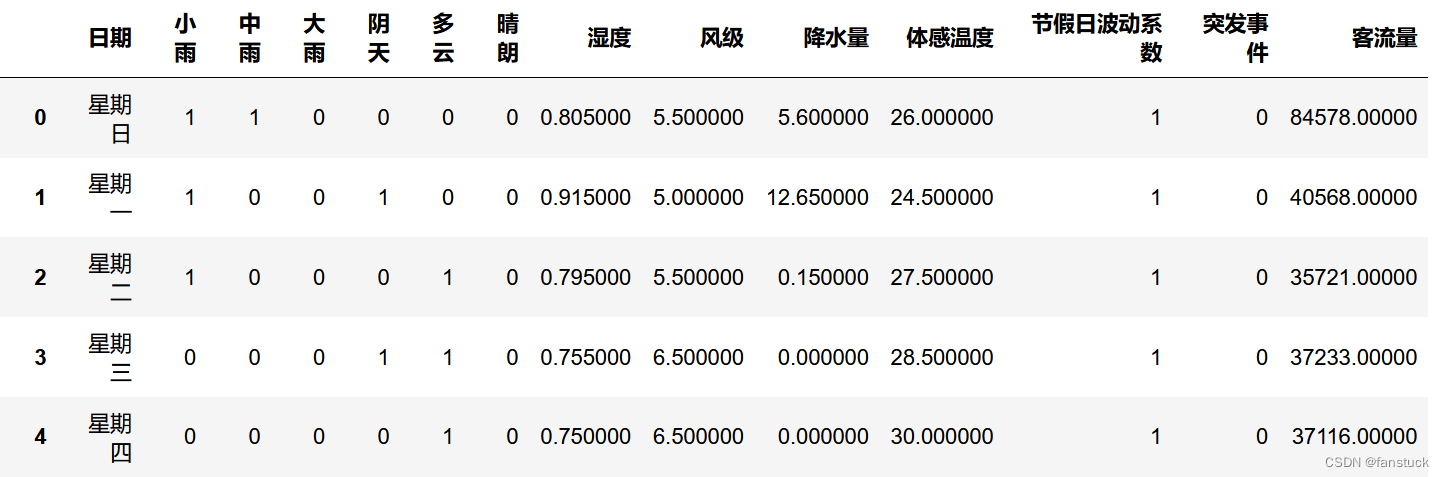• Series.plot.box()
• DataFrame.plot.box()
• DataFrame.boxplot()

``````df_flow_mark[['风级','降水量']].plot.box()
``````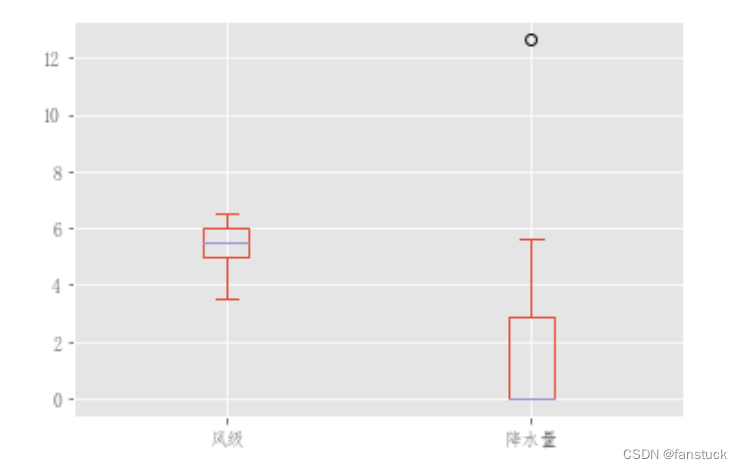``````color = {
"boxes": "DarkGreen",
"whiskers": "DarkOrange",
"medians": "DarkBlue",
"caps": "Gray",
}
df_flow_mark[['风级','降水量']].plot.box(color=color, sym="r+")
``````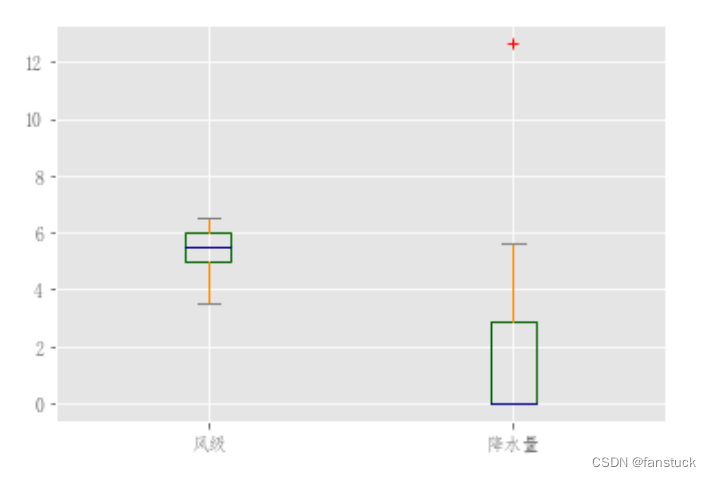``````df = pd.DataFrame(np.random.rand(10, 5), columns=["A", "B", "C", "D", "E"])
color = {
"boxes": "DarkGreen",
"whiskers": "DarkOrange",
"medians": "DarkBlue",
"caps": "Gray",
}
df.plot.box(color=color, sym="r+")
``````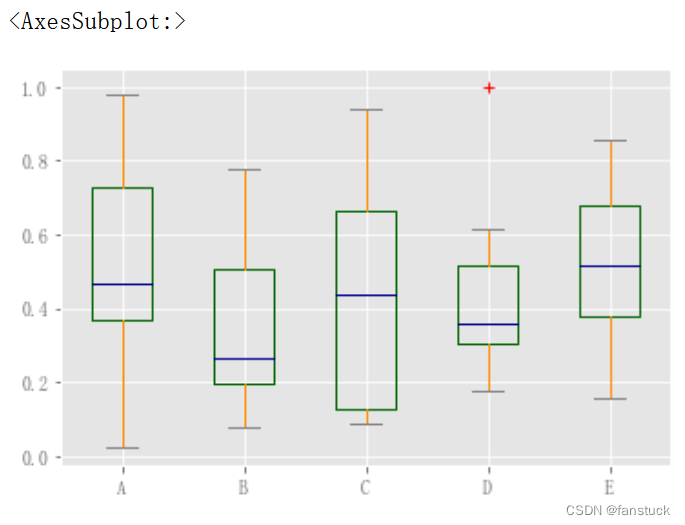``````df.plot.box(vert=False, positions=[1, 4, 5, 6, 8])
``````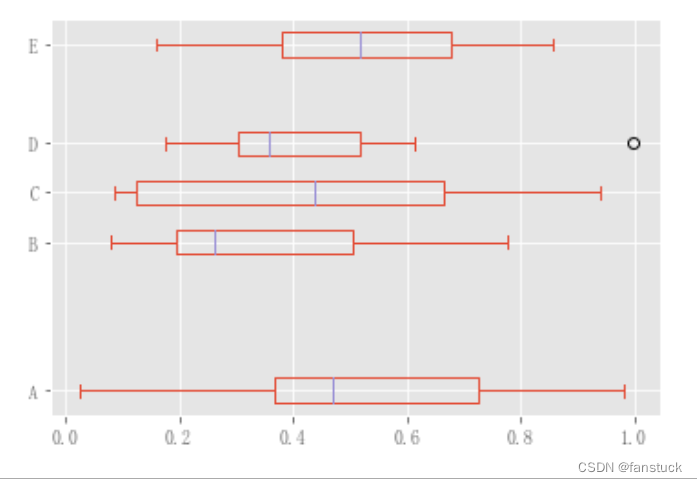``````df.boxplot()
``````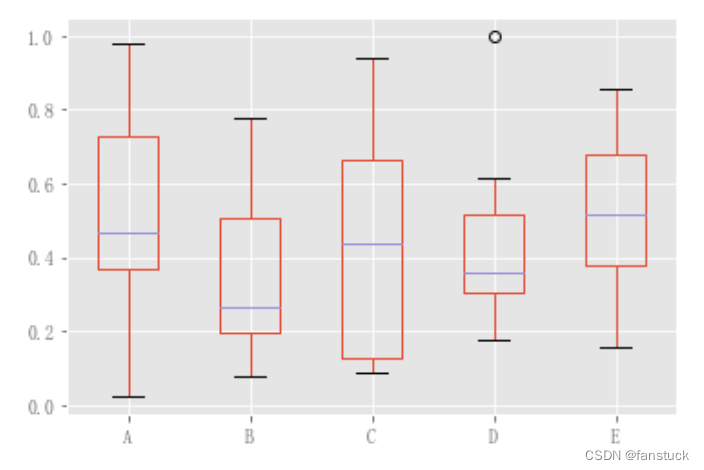``````df = pd.DataFrame(np.random.rand(10, 2), columns=["Col1", "Col2"])
df["X"] = pd.Series(["A", "A", "A", "A", "A", "B", "B", "B", "B", "B"])
plt.figure();
bp = df.boxplot(by="X")
``````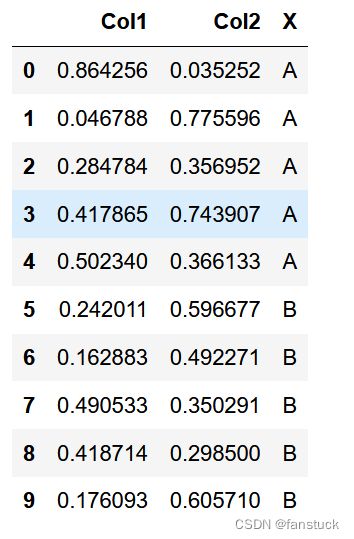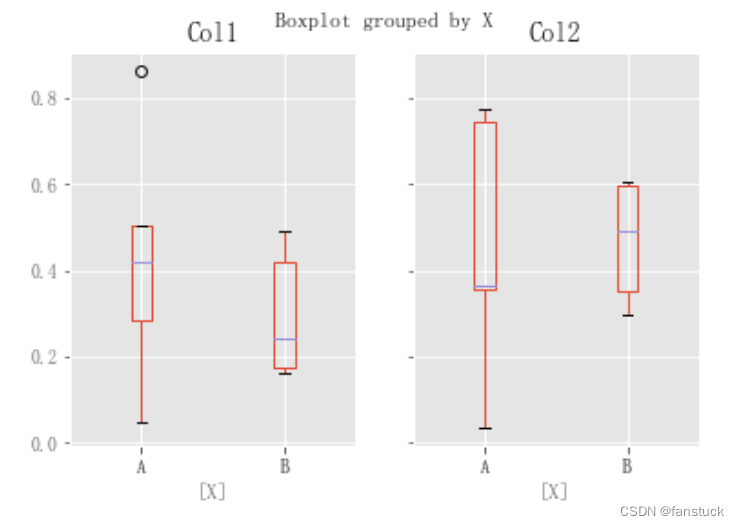``````df = pd.DataFrame(np.random.rand(10, 3), columns=["Col1", "Col2", "Col3"])
df["X"] = pd.Series(["A", "A", "A", "A", "A", "B", "B", "B", "B", "B"])
df["Y"] = pd.Series(["A", "B", "A", "B", "A", "B", "A", "B", "A", "B"])
plt.figure();
bp = df.boxplot(column=["Col1", "Col2"], by=["X", "Y"])
``````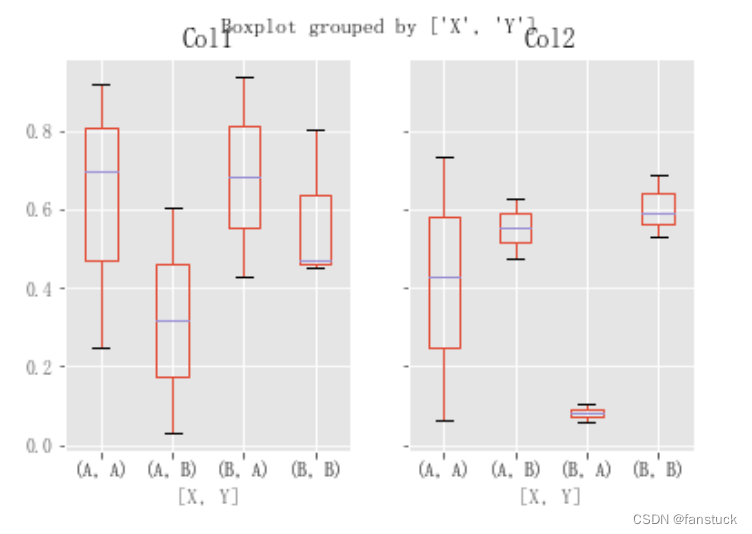``````df = pd.DataFrame(np.random.rand(10, 3), columns=["Col1", "Col2", "Col3"])
df["X"] = pd.Series(["A", "A", "A", "A", "A", "B", "B", "B", "B", "B"])
plt.figure()
bp = df.plot.box(columns=["Col1", "Col2"], by="X")
``````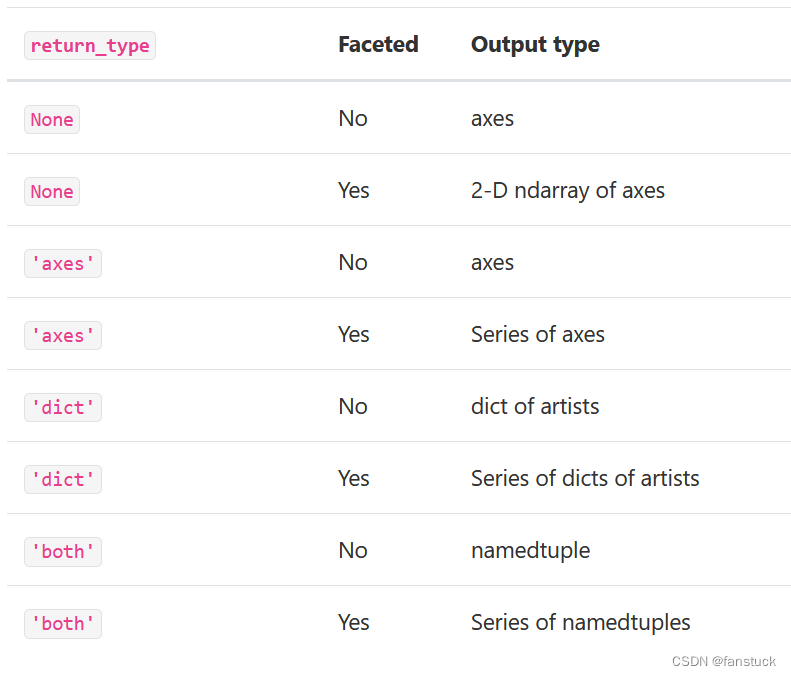``````np.random.seed(1234)
df_box = pd.DataFrame(np.random.randn(50, 2))
df_box["g"] = np.random.choice(["A", "B"], size=50)
df_box.loc[df_box["g"] == "B", 1] += 3
bp = df_box.boxplot(by="g")
``````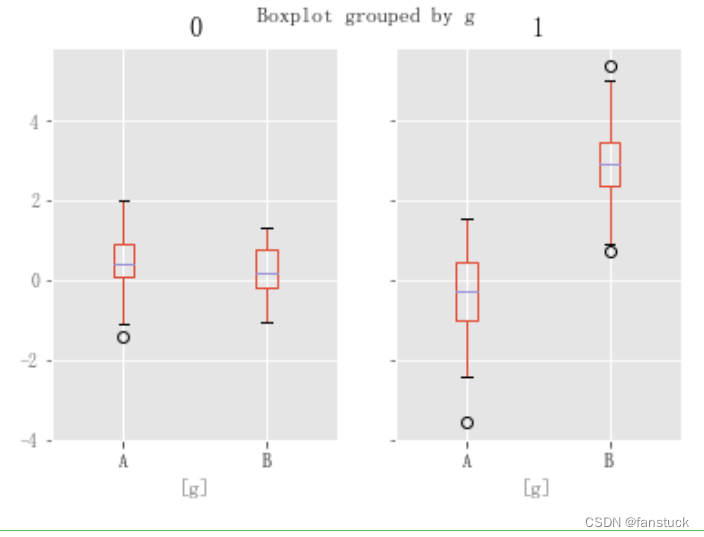``````bp = df_box.groupby("g").boxplot()
``````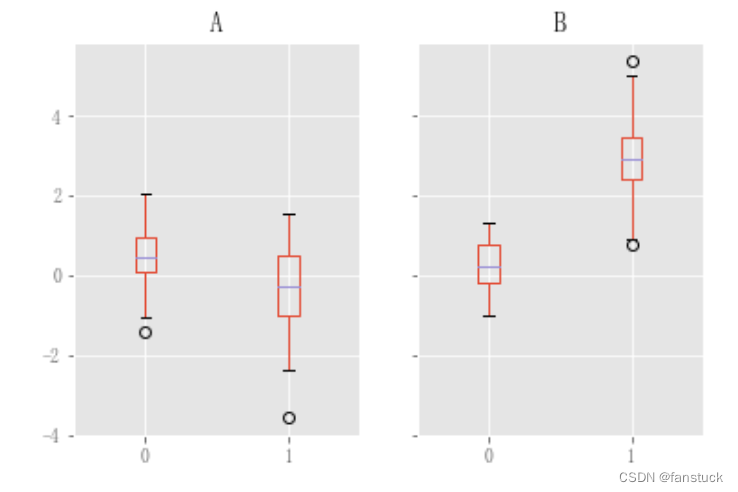## 二、面积填充图

``````df = pd.DataFrame(np.random.rand(10, 4), columns=["a", "b", "c", "d"])
df.plot.area();
``````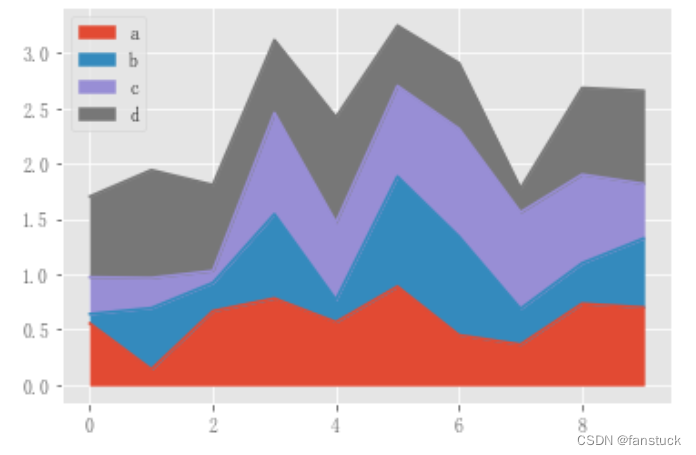``````df.plot.area(stacked=False);
``````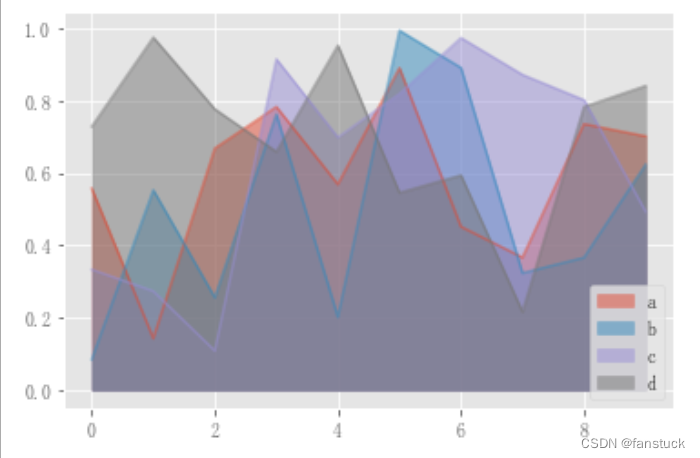## 三、散点图

``````df_flow_mark.plot.scatter(x='日期',y='客流量')
``````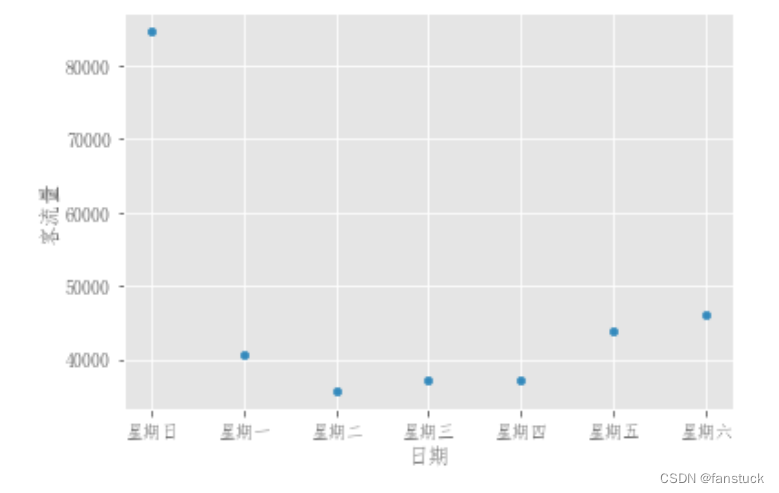``````df = pd.DataFrame(np.random.rand(50, 4), columns=["a", "b", "c", "d"])
df["species"] = pd.Categorical(
["setosa"] * 20 + ["versicolor"] * 20 + ["virginica"] * 10
)
ax = df.plot.scatter(x="a", y="b", color="DarkBlue", label="Group 1")
df.plot.scatter(x="c", y="d", color="DarkGreen", label="Group 2", ax=ax);
``````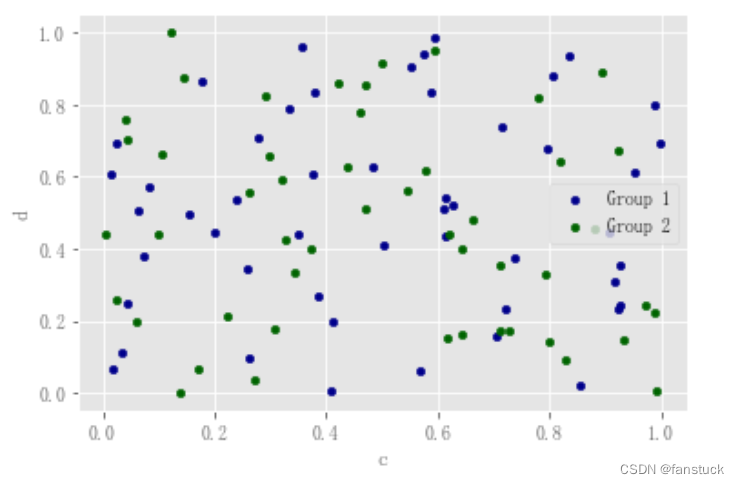``````df.plot.scatter(x="a", y="b", c="c", s=50);
``````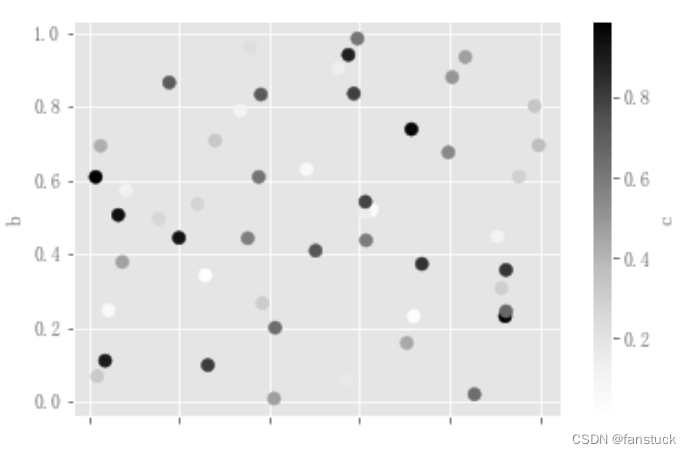``````df.plot.scatter(x="a", y="b", c="species", cmap="viridis", s=50);
``````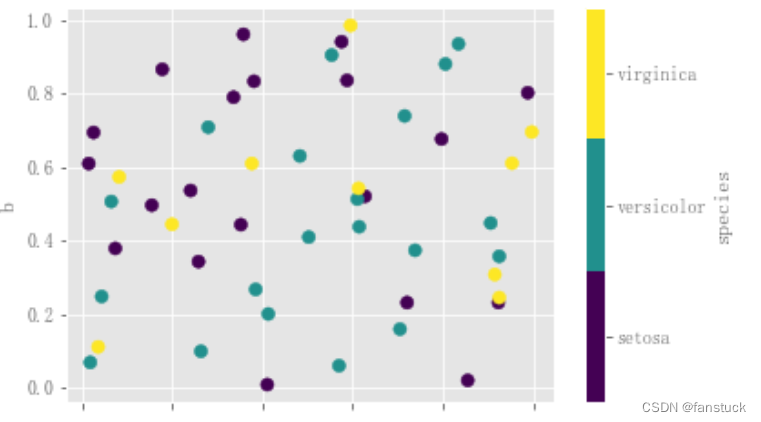``````df_flow_mark.plot.scatter(x='日期',y='客流量',s=df_flow_mark['湿度']*200)
``````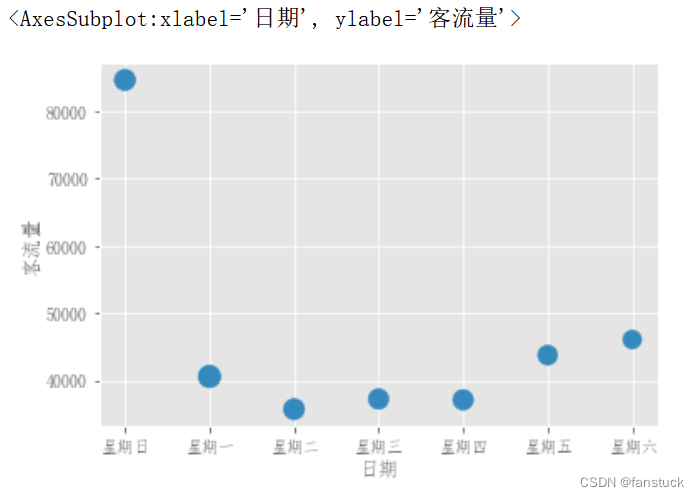### “Pandas数据分析：快速图表可视化各类操作详解+实例代码(二)”的评论:

##### 关于作者##### overfit同步小助手